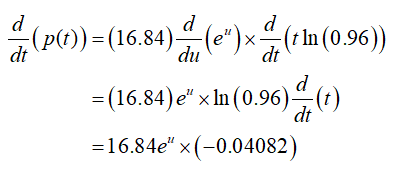Differentiate the following function:P(t)=16.84 * (0.96)^tEnter the derivative here:

Question

Differentiate the following function:
P(t)=16.84 * (0.96)^t

Enter the derivative here:

Step 1

Given:

The function p(t) = (16.84)(0.96)t.

Step 2

Calculation:

Differentiate p(t) with respect to t.

Step 3

Let f = eu and u = t(ln(0.96))....

Want to see the full answer?

See Solution

Want to see this answer and more?

Our solutions are written by experts, many with advanced degrees, and available 24/7

See Solution
Tagged in

Derivative